Most Affordable JEE | NEET | 8,9,10 Preparation by Kota's Top IITian Doctor Faculties

# Chemical Equilibrium - JEE Mains Previous Year QuestionsJEE Main Previous Year Papers Questions of Chemistry With Solutions are available at eSaral.   Simulator   Previous Years AIEEE/JEE Mains Questions
Q. A vessel at 1000 K contains $\mathrm{CO}_{2}$ with a pressure of 0.5 atm. Some of the $\mathrm{CO}_{2}$ is converted into CO on the addition of graphite. If the total pressure at equilibrium is 0.8 atm, the value of K is :- (1) 0.3 atm (2) 0.18 atm (3) 1.8 atm (4) 3 atm [AIEEE-2011]
Ans. (3)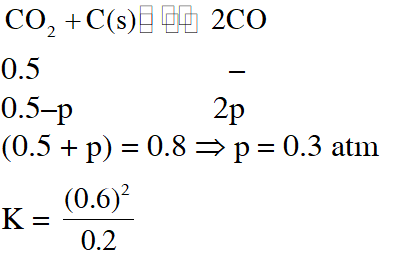Q. The equilibrium constant $\left(\mathrm{K}_{\mathrm{C}}\right)$ for the reaction $\mathrm{N}_{2}(\mathrm{g})+\mathrm{O}_{2}(\mathrm{g}) \longrightarrow 2 \mathrm{NO}(\mathrm{g})$ at temperature T is $4 \times 10^{-4}$ The value of $\mathrm{K}_{\mathrm{c}}$ for the reaction. $\mathrm{NO}(\mathrm{g}) \longrightarrow 1 / 2 \mathrm{N}_{2}(\mathrm{g})+1 / 2 \mathrm{O}_{2}(\mathrm{g})$ at the same temperature is :- (1) 50.0 (2) 0.02 (3) $2.5 \times 10^{2}$ (4) $4 \times 10^{-4}$ [AIEEE-2012]
Ans. (1) $\mathrm{K}_{\mathrm{c}}=\frac{1}{\sqrt{\mathrm{K}_{\mathrm{c}}}}$
Q. 8 mol of $\mathrm{AB}_{3}(\mathrm{g})$ are introduced into a 1.0 $\mathrm{d} \mathrm{m}^{3}$ vessel. If it dissociates as $2 \mathrm{AB}_{3}(\mathrm{g}) \square \quad \mathrm{A}_{2}(\mathrm{g})+3 \mathrm{B}_{2}(\mathrm{g})$ At equilibrium, 2mol of $\mathrm{A}_{2}$ are found to be present. The equilibrium constant of this reaction is :- (1) 36 (2) 3 (3) 27 (4) 2 [JEE-MAINS(online)-2012]
Ans. (3)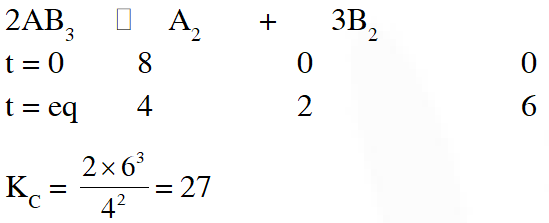Q. The value of Kp for the equilibrium reaction $\mathrm{N}_{2} \mathrm{O}_{4}(\mathrm{g}) \square 2 \mathrm{NO}_{2}(\mathrm{g})$ is 2 The percentage dissociation of $\mathrm{N}_{2} \mathrm{O}_{4}(\mathrm{g})$ at a pressure of 0.5 atm is (1) 71 (2) 50 (3) 88 (4) 25 [JEE-MAINS(online)-2012]
Ans. (1) $\mathrm{K}_{\mathrm{p}}=\frac{(2 \alpha)^{2}}{(1-\alpha)} \times \frac{0.5}{(1+\alpha)}$
Q. $\mathrm{K}_{1}, \mathrm{K}_{2}$ and $\mathrm{K}_{3}$ are the equilibrium constants of the following reactions (I), (II) and (III), respectively (I) $\mathrm{N}_{2}+2 \mathrm{O}_{2} \square 2 \mathrm{NO}_{2}$ (II) $2 \mathrm{NO}_{2} \square \mathrm{N}_{2}+2 \mathrm{O}_{2}$ (III) $\mathrm{NO}_{2} \square \frac{1}{2} \mathrm{N}_{2}+\mathrm{O}_{2}$ The correct relation from the following is : (1) $\mathrm{K}_{1}=\sqrt{\mathrm{K}_{2}}=\mathrm{K}_{3}$ (2) $\mathrm{K}_{1}=\frac{1}{\mathrm{K}_{2}}=\frac{1}{\mathrm{K}_{3}}$ $(3) \mathrm{K}_{1}=\frac{1}{\mathrm{K}_{2}}=\mathrm{K}_{3}$ (4)$\mathrm{K}_{1}=\frac{1}{\mathrm{K}_{2}}=\frac{1}{\left(\mathrm{K}_{3}\right)^{2}}$ [JEE-MAINS(online)-2012]
Ans. (4) Fact
Q. One mole of $\mathrm{O}_{2}(\mathrm{g})$ and two moles of SO2(g) were heated in a closed vessel of one litre capacity at 1098 K. At equilibrium 1.6 moles of $\mathrm{SO}_{3}$ (g) were found. The equilibrium constant $\mathbf{K}_{C}$ of the reaction would be :- (1) 60 (2) 80 (3) 30 (4) 40 [JEE-MAINS(online)-2012]
Ans. (2)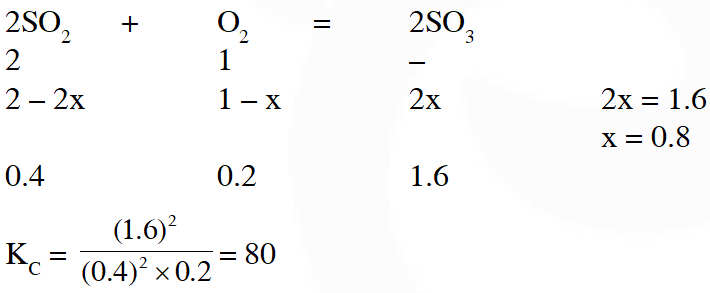Q. $\mathrm{N}_{2}(\mathrm{g})+3 \mathrm{H}_{2}(\mathrm{g}) \square 2 \mathrm{NH}_{3}(\mathrm{g}), \mathrm{K}_{1}$ $\mathrm{N}_{2}(\mathrm{g})+\mathrm{O}_{2}(\mathrm{g}) \square 2 \mathrm{NO}(\mathrm{g}), \mathrm{K}_{2} \quad(\mathrm{B})$ $\mathrm{H}_{2}(\mathrm{g})+\frac{1}{2} \mathrm{O}_{2}(\mathrm{g}) \square \mathrm{H}_{2} \mathrm{O}(\mathrm{g}), \mathrm{K}_{3} \quad(\mathrm{C})$ The equation for the equilibrium constant of the reaction $2 \mathrm{NH}_{3}(\mathrm{g})+\frac{5}{2} \mathrm{O}_{2}(\mathrm{g}) \square 2 \mathrm{NO}(\mathrm{g})+3 \mathrm{H}_{2} \mathrm{O}(\mathrm{g}),\left(\mathrm{K}_{4}\right)$ in terms of $\mathrm{K}_{1}, \mathrm{K}_{2}$ and $\mathrm{K}_{3}$ is : (1) $\frac{\mathrm{K}_{1} \mathrm{K}_{3}^{2}}{\mathrm{K}_{2}}$ (2) $\frac{\mathrm{K}_{2} \mathrm{K}_{3}^{3}}{\mathrm{K}_{1}}$ (3) $\frac{\mathrm{K}_{1} \mathrm{K}_{2}}{\mathrm{K}_{3}}$ (4) $\mathrm{K}_{1} \mathrm{K}_{2} \mathrm{K}_{3}$ [JEE-MAINS(online)-2013]
Ans. (2) Fact
Q. In reaction $\mathrm{A}+2 \mathrm{B} \square 2 \mathrm{C}+\mathrm{D}$, initial concentration of B was 1.5 times of |A|, but at equilibrium the concentrations of A and B became equal. The equilibrium constant for the reaction is (1)4 (2) 6 (3) 12 (4) 8 [JEE-MAINS(online)-2013]
Ans. (1)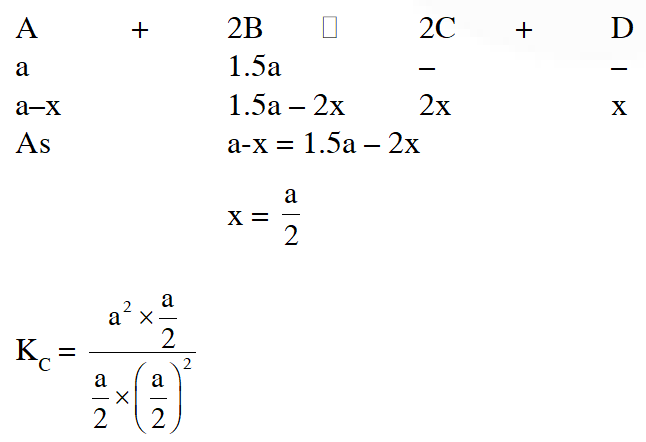$\mathrm{K}_{\mathrm{c}}=4$
Q. For the decomposition of the compound, represented asIf the reaction is started with 1 mol of the compound, the total pressure at equilibrium would be (1) $38.8 \times 10^{-2}$ atm (2) $1.94 \times 10^{-2}$ atm (3) $5.82 \times 10^{-2}$ atm (4) $7.66 \times 10^{-2}$ atm [JEE-MAINS(online)-2014]
Ans. (3)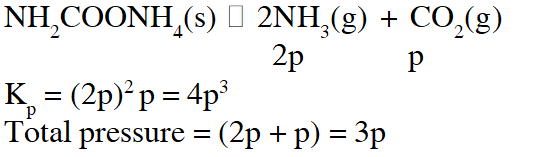Q. For the reaction $\mathrm{SO}_{2(\mathrm{g})}+\frac{1}{2} \mathrm{O}_{2(\mathrm{g})} \square \mathrm{SO}_{3(\mathrm{g})},$ if $\mathrm{K}_{\mathrm{p}}=\mathrm{K}_{\mathrm{C}}(\mathrm{RT})^{\mathrm{x}}$ where the symbols have usual meaning then the value of x is : (assuming ideality) ( 1)$\frac{1}{2}$ ( 2) 1 (3) –1 $(4)-\frac{1}{2}$ [JEE-MAINS 2014]
Ans. (4) $\mathrm{K}_{\mathrm{p}}=\mathrm{K}_{\mathrm{C}}(\mathrm{RT})^{-\frac{1}{2}}$
Q. The equilibrium constants at 298 K for a reaction $\mathrm{A}+\mathrm{B} \rightleftharpoons \mathrm{C}+\mathrm{D}$ is 100 If the initial concentration of all the four species were 1 M each, then equilibrium concentration of D (in mol $\mathrm{L}^{-1}$) will be : (1) 1.182 (2) 0.182 (3) 0.818 (4) 1.818 [JEE-Mains 2016]
Ans. (4)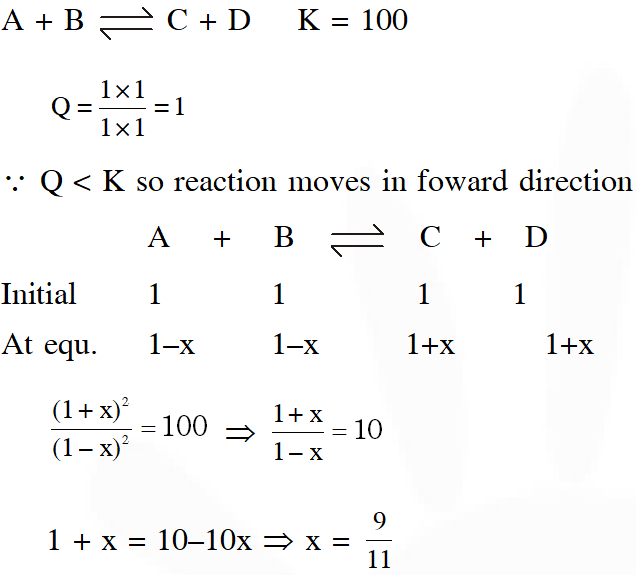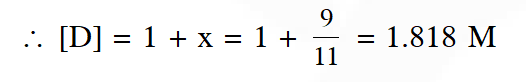Pratik
Sept. 16, 2022, 9:35 a.m.
More questions
Hagu
Oct. 8, 2021, 7:19 a.m.
Proper solution
ram
Aug. 22, 2021, 12:05 p.m.
try some hard questions
A
July 10, 2021, 3:19 p.m.
Fuck u
Dhruva
May 14, 2021, 8:54 p.m.
awesome
Om
Feb. 7, 2021, 12:15 p.m.
Thanks for the list
Monu
Dec. 28, 2020, 8:58 a.m.
The answer is k1=1/k2=1/√k3 not (k3)^2
praful
Dec. 6, 2020, 4:08 p.m.
its too good
Nov. 10, 2020, 11:21 p.m.
thank you in other websites the questions are simple but here they are tricky and good solution releaving method is excellent thank you very
L4WUDU
Nov. 5, 2020, 9:56 p.m.
R4
Nov. 3, 2020, 7:34 p.m.
please give a little harder questions
Nenavath Shiva Naik
Oct. 20, 2020, 9:51 p.m.
Better to improve standard of questions
Fate
Oct. 3, 2020, 10:26 p.m.
Great job
Nikki
Sept. 30, 2020, 11:50 a.m.
Thank you very much so good questions
Angel
Aug. 24, 2020, 5:39 p.m.
Good
Ganesh
Aug. 1, 2020, 6:23 a.m.
Nice But make questions on degree of dissociation Make some what difficult question. I think u can do the best I like this esaral To continue in it please post tough questions with solutions
pranith
June 26, 2020, 4:47 p.m.
tnq u bro
Akhil
May 23, 2020, 2:17 p.m.
Chill bro chill
Akhil
May 23, 2020, 2:16 p.m.
Chill bro
Harshitha
May 14, 2020, 6:39 a.m.
Thanku very much
Sai tharun
May 11, 2020, 9:03 p.m.
Thank u
Praful ghait
May 3, 2020, 4:50 p.m.
Can you give me test series for imucet exam
Koushika
April 14, 2020, 6:21 p.m.
Thank you so much